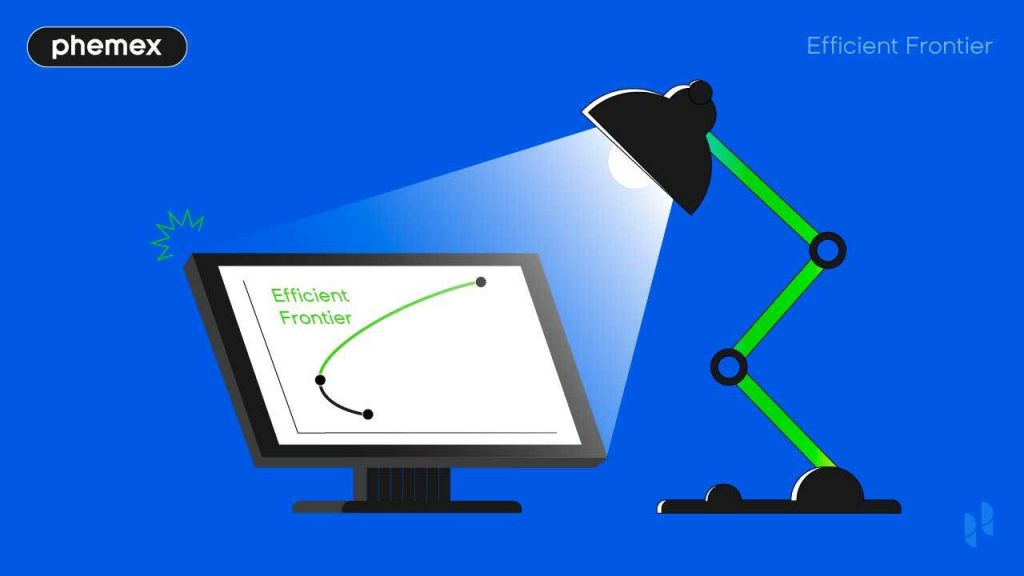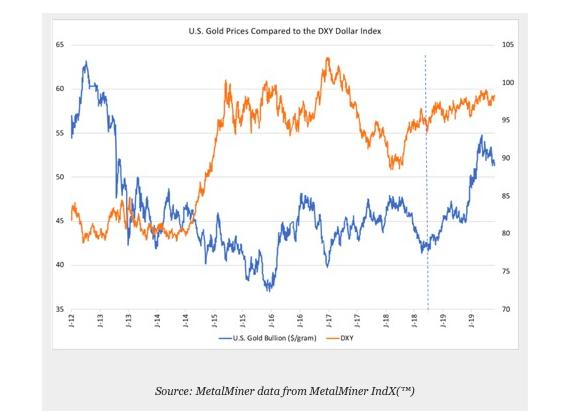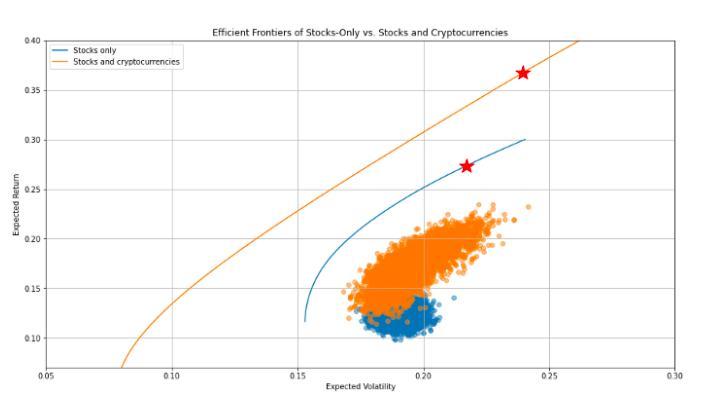Academy > Technical Analysis > What is The Efficient Frontier: Key to Profitable Investing >

# What is The Efficient Frontier: Key to Profitable Investing

2021-12-03 11:30:16

Investing money is risky business, but it can also be highly rewarding. To increase chances of profitability, various economists and financial analysts have offered up their theories and methods to find a way to invest with maximum reward and minimum risk. One such economist was Dr. Harry Markowitz, who in 1952 came up with the efficient frontier concept as a cornerstone of his modern portfolio theory (MPT).## Understanding Efficient Frontier Through Modern Portfolio Theory

To understand what the efficient frontier is, one must first understand what modern portfolio theory (MPT) is. Before MPT theory, the generally accepted rule was that the amount of reward was linear with the level of risk, meaning that the higher the risk (also known as standard deviation), the higher the reward (also known as expected return), and vice versa. This can be seen by the graph below:

Generally, bonds were considered low risk, low reward, while stocks were considered high risk, high reward. This meant that an investor could choose their investment type based on their tolerance to risk. It also meant that those who were wealthier stood a better chance of getting more returns on their investments faster, as they had a higher tolerance to risk.

However, in 1952, American economist Dr. Harry Markowitz proposed that by diversifying a portfolio, an investor could in fact find a sweet spot where they could earn high rewards while not being subject to unacceptable levels of risk. This was known as MPT, and in many ways, it meant that investors with less to lose had a better chance of earning high returns. For those with a lower tolerance to risk than the MPT may be able to offer, however, there is also post-modern portfolio theory (PMPT).

Markowitz argued that by diversifying a portfolio, the risk-to-return trade off was no longer linear, but curved, meaning that you could have the same level, or at least a lower level, of risk but with a higher level of return. This curved line is known as the “efficient frontier.”

It must be stated that diversifying does not mean buying up assets that are different to each other, rather it means buying assets that have a negative correlation, i.e., their values do not follow each other. After all, if both assets are positively correlated — their values follow each other — then when making a loss, the loss would be made on both assets; something which would be counterproductive. Diversification works because the loss in one asset is likely to be offset by a gain in the other asset.An example of the negative correlation of two stocks (US gold bullion and DXY dollar index). (Source: agmetalminer.com)

## What Is the Efficient Frontier?

The efficient frontier, also known as a portfolio frontier, is the line that dictates which portfolios are the most efficient (lower risk to higher return), and how efficient they are compared to one another. Those portfolios — which are combinations of two different shares — that are closest to the efficient frontier, are those that are the most efficient. Those further below it will be either high risk/low reward, or low risk but equally low reward.

This is clearly illustrated in the graph below, with the efficient frontier being the XY curve:

Investors are encouraged to buy the combinations of two shares, known as a portfolio, that would place their investment on, or as close as possible to, the efficient frontier. According to MPT, this will increase their chances of making good returns while tolerating a lower risk. In addition, by being able to visualize the level of risk and return of each portfolio, an investor can easily decide on the level of risk they want to accept.

• In the graph above, if an investor wants a low-risk investment, they will choose a portfolio that is on the efficient frontier but closer to the Y point.
• In the same graph, if an investor wants the possibility of higher returns, and can tolerate higher risk, they will choose a portfolio on the efficient frontier but closer to the X point.
• Those portfolios closest to the Z point are considered the worst options, containing a combination of shares that would not only yield low returns, but also leave the investor open to unnecessary risk.

## How Is the Efficient Frontier Calculated?

Dr. Markowitz’s argument was that if he invested 100% in one asset, then if there was a bear market, he would look like a fool. Likewise, if he didn’t invest, and the market turned bullish, he would again look like a fool. To counter this, he concluded that through mathematics, one could calculate the right percentage to invest in two or more negatively correlated assets, so as to mitigate the loss in one with the return from another.

Once the two assets are chosen, their combined expected return and standard deviation (risk) need to be calculated. This would then place them on the chart as a dot, and if that dot is close to the efficient frontier, then it would be considered a good investment.

This process is repeated (an automated process) for all the investment pairs an investor would like to look at. For example, if an investor chooses to look at possible investments in 6 different cryptocurrencies or stocks, then there will be a total of 720 dots on the graph: one dot for each possible combination.

The efficient frontier line itself is simply the line that follows the best possible portfolio combinations with regards to their risk-to-return.### How Are the Expected Return and Standard Deviation (Risk) of a Portfolio Calculated?

The above may seem simple, but it is important to understand exactly how the return and risk of a portfolio is calculated. When investing money, there is always a level of risk, even if you choose a portfolio at the point of the efficient frontier with the lowest risk. Thus, it’s wise to know what is behind the calculations of the efficient frontier asset allocation, which, if you follow MPT, will be where you invest your hard-earned money.

• The expected return of a portfolio is calculated by calculating the expected returns of each asset, based on the amount invested, and then adding them together.
• An example would be if an individual were to invest a total of \$5,000, with \$4,000 allocated to asset A, and \$1,000 allocated to asset B. If the expected return for asset A is 10% and the expected return on asset B is 3%, then the whole portfolio’s expected return would be calculated as follows (with the resulting 8.6% being the expected return on the whole portfolio):

((\$4,000 / \$5,000) × 10%) + ((\$1,000 / \$5,000) × 3%) = (0.8 × 10%) + (0.2 × 3%) = 8.6%

• The standard deviation, or risk, of a portfolio is calculated based on how much movement there is in the price, i.e., its volatility. As with the expected return of an asset, the standard deviation considers both assets separately and then adds them together. However, with standard deviation, the correlation must also be considered. So, in total there are three aspects to consider when calculating the risk of a portfolio:
• The standard deviation of each asset independently
• The amount being invested in each asset
• The correlation (either negative or positive) between the assets

## The Efficient Frontier in Crypto

As with any trading pairs, the efficient frontier can also be applied in the crypto space. In the past, as most cryptocurrencies were positively correlated with Bitcoin (BTC), its usefulness may well have been debated. Today, however, with the rise of decentralized finance (DeFi) the crypto market has diversified, with different tokens and coins offering a vast array of services, and all with their own value patterns.

This diversification means that using MPT and its efficient frontier certainly has its uses when calculating where to allocate one’s investments in crypto. However, it is still important to diversify in the crypto space, for example, investing solely in stable coins is unlikely to yield the results one wants.

A good idea can be to invest in both cryptocurrency and stocks, as these are quite often negatively correlated. When consumers flock to cryptocurrencies, for example, this quite often indicates a lack of trust in established and centralized financial institutions.

Jonas Benner from “Towards Data Science” has a good analysis on using the efficient frontier for both stocks and crypto. He uses historical data (2015 to 2020) to explore the correlation between the two types of assets and how combining the two would affect a portfolio. His findings show that by combining the two, one can achieve a much better risk-to-return ratio, as can be seen below. However, it must be noted that cryptocurrencies are an exceedingly volatile asset and thus his research may not depict today’s financial realities. It is therefore of paramount importance that each investor do their own research.Jonas Benner’s analysis on combining stocks and crypto showed that the possibility for higher returns was increased while the risk remained the same or only increased by a small amount. Orange displays portfolios that combine stocks and crypto while blue are portfolios without crypto. (Source: towardsdatascience.com)

## Is the Efficient Erontier Useful?

The efficient frontier has been used by probably every serious investor since the 1950s — Dr. Markowitz even won the Nobel prize for his work on MPT. Interestingly, however, the very same Dr. Markowitz stated in an interview with MIT Laboratory for Financial Engineering in 2017 that he would not recommend using the efficient frontier in the same way as he did in 1952 for today’s market.

In 1952, Dr. Markowitz advised caution, creating a portfolio pair of a low-risk asset, such as a bond, with a higher risk asset, such as a stock. Today, he advises to maximize profitability by embracing a higher risk — but not an exceedingly high one. This is because today’s technology is far superior to that available in 1952, including optimizers, and much more sophisticated prediction and analysis techniques. This bodes well for using the efficient frontier with crypto, as not only is it often a high risk/high return asset, but there are multiple tools that can be used to optimize investment strategies and calculate allocation percentages.

Dr. Markowitz’s more up-to-date views on MPT tell us that the efficient frontier is still a very useful tool to determine the perfect portfolio. His comments on embracing assets with higher risk/higher returns (within reason) may also tell us how to use this tool with cryptocurrencies. Perhaps then, combining cryptocurrencies — which are highly volatile but often high reward — with assets with a lower risk, but not too low risk as not to give good returns, is the best way to use this tool for maximum profit.

Phemex | Break Through, Break FreeRegister to get \$180 Welcome Bonus!
Email